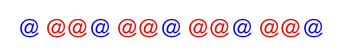【每日一题0110】手串计数已知一个手串是由红色和蓝色珠子搭配构成的，并且每两个蓝色珠子之间夹着连续的2个红色珠子。def solution(num: int) -> int:

assert solution(1) == 0
assert solution(3) == 4
assert solution(5) == 8
def solution(num: int) -> int:
if num < 2:
return 0
red = (num-1) * 2
return red

assert solution(1) == 0
assert solution(3) == 4
assert solution(5) == 8
1 Like
def solution(num: int) -> int:
if num < 2:
return 0
return (num - 1) * 2
1 Like
def solution(num: int) -> int:
return 0 if num < 2 else (num-1)*2

def solution0110(num: int) -> int:
# 已知一个手串是由红色和蓝色珠子搭配构成的，并且每两个蓝色珠子之间夹着连续的2个红色珠子
# 请编写一个函数，输入一个蓝色珠子的数量num，请求出红色珠子的个数。如果输入的数字小于2则返回0

return (num-1)*2 if num >=2 else 0
def solution16(num:int):
if num<2:
return 0
else:
return (num-1)*2
assert solution16(1) == 0
assert solution16(3) == 4
assert solution16(5) == 8
def solution(num: int) -> int:
return (num - 1) * 2 if num > 1 else 0
def solution(num: int) -> int:
return 0 if num<2 else (num-1)*2

def solution(num: int) → int:
return （num-1）*2

def solution(num: int) -> int:
return 2*(num-1) if num >1 else 0

assert solution(1) == 0
assert solution(3) == 4
assert solution(5) == 8
def solution(num: int) -> int:
return 0 if num < 2 else (num - 1) * 2

assert solution(1) == 0
assert solution(3) == 4
assert solution(5) == 8
def solution(num: int) -> int:
return (num - 1) * 2 if num > 0 else 0

assert solution(1) == 0
assert solution(3) == 4
assert solution(5) == 8